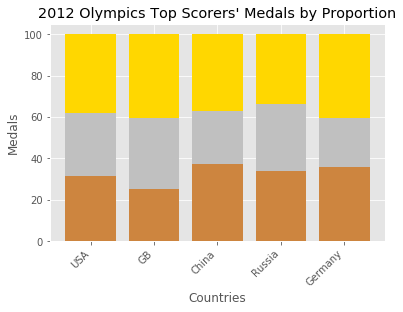# Bar Charts in Matplotlib

Bar charts are used to display values associated with categorical data.

The plt.bar function, however, takes a list of positions and values, the labels for x are then provided by plt.xticks().

In :
import matplotlib.pyplot as plt
%matplotlib inline
plt.style.use('ggplot')

x = ['Nuclear', 'Hydro', 'Gas', 'Oil', 'Coal', 'Biofuel']
energy = [5, 6, 15, 22, 24, 8]

x_pos = [i for i, _ in enumerate(x)]

plt.bar(x_pos, energy, color='green')
plt.xlabel("Energy Source")
plt.ylabel("Energy Output (GJ)")
plt.title("Energy output from various fuel sources")

plt.xticks(x_pos, x)

plt.show()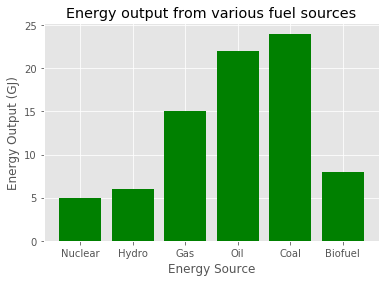### Error bars

We can extend the above with error bars as follows

In :
x = ['Nuclear', 'Hydro', 'Gas', 'Oil', 'Coal', 'Biofuel']
energy = [5, 6, 15, 22, 24, 8]
variance = [1, 2, 7, 4, 2, 3]

x_pos = [i for i, _ in enumerate(x)]

plt.bar(x_pos, energy, color='green', yerr=variance)
plt.xlabel("Energy Source")
plt.ylabel("Energy Output (GJ)")
plt.title("Energy output from various fuel sources")

plt.xticks(x_pos, x)

plt.show()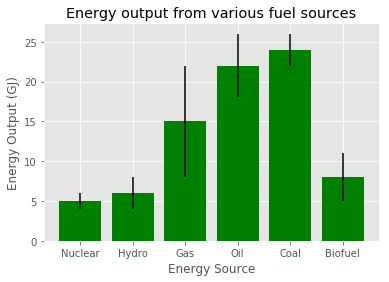### Horizontal Bar Chart

We can show the exact same chart horizontally using plt.barh()

In :
x = ['Nuclear', 'Hydro', 'Gas', 'Oil', 'Coal', 'Biofuel']
energy = [5, 6, 15, 22, 24, 8]
variance = [1, 2, 7, 4, 2, 3]

x_pos = [i for i, _ in enumerate(x)]

plt.barh(x_pos, energy, color='green', xerr=variance)
plt.ylabel("Energy Source")
plt.xlabel("Energy Output (GJ)")
plt.title("Energy output from various fuel sources")

plt.yticks(x_pos, x)

plt.show()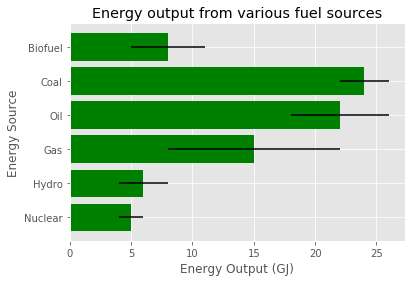### Bar Chart with Multiple X’s

To include multiple X values on the same chart, we can reduce the width of the bars and then place the indices one bar’s width further from the y axis.

In :
import numpy as np

N = 5
men_means = (20, 35, 30, 35, 27)
women_means = (25, 32, 34, 20, 25)

ind = np.arange(N)
width = 0.35
plt.bar(ind, men_means, width, label='Men')
plt.bar(ind + width, women_means, width,
label='Women')

plt.ylabel('Scores')
plt.title('Scores by group and gender')

plt.xticks(ind + width / 2, ('G1', 'G2', 'G3', 'G4', 'G5'))
plt.legend(loc='best')
plt.show()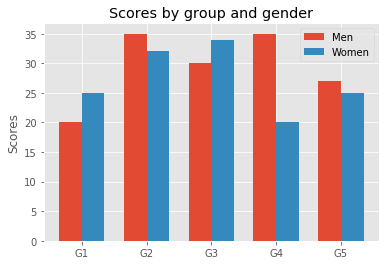### Stacked Bar Chart

With stacked bar charts we need to provide the parameter bottom, this informs matplotlib where the bar should start from, so we will add up the values below.

In :
countries = ['USA', 'GB', 'China', 'Russia', 'Germany']
bronzes = np.array([38, 17, 26, 19, 15])
silvers = np.array([37, 23, 18, 18, 10])
golds = np.array([46, 27, 26, 19, 17])
ind = [x for x, _ in enumerate(countries)]

plt.bar(ind, golds, width=0.8, label='golds', color='gold', bottom=silvers+bronzes)
plt.bar(ind, silvers, width=0.8, label='silvers', color='silver', bottom=bronzes)
plt.bar(ind, bronzes, width=0.8, label='bronzes', color='#CD853F')

plt.xticks(ind, countries)
plt.ylabel("Medals")
plt.xlabel("Countries")
plt.legend(loc="upper right")
plt.title("2012 Olympics Top Scorers")

plt.show()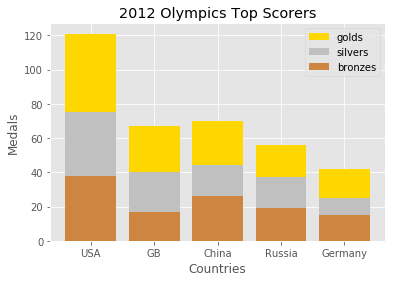If we wanted to view the same bar charts but as a proportion of the total medals won by that country, we can do the following:

In :
total = bronzes + silvers + golds
proportion_bronzes = np.true_divide(bronzes, total) * 100
proportion_silvers = np.true_divide(silvers, total) * 100
proportion_golds = np.true_divide(golds, total) * 100

plt.bar(ind, proportion_golds, width=0.8, label='golds', color='gold', bottom=proportion_bronzes+proportion_silvers)
plt.bar(ind, proportion_silvers, width=0.8, label='silvers', color='silver', bottom=proportion_bronzes)
plt.bar(ind, proportion_bronzes, width=0.8, label='bronzes', color='#CD853F')

plt.xticks(ind, countries)
plt.ylabel("Medals")
plt.xlabel("Countries")
plt.title("2012 Olympics Top Scorers' Medals by Proportion")
plt.ylim=1.0

# rotate axis labels
plt.setp(plt.gca().get_xticklabels(), rotation=45, horizontalalignment='right')

plt.show()